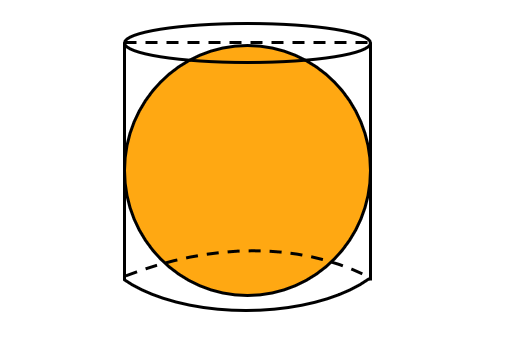# Surface Areas Cylinder & Sphere | Exercise 13.4 | Q9

###### CBSE Class 9 Maths | NCERT Solution to Exercise

Question 9: A right circular cylinder just encloses a sphere of radius r. Find(i) surface area of the sphere
(ii) curved surface area of the cylinder,
(iii) ratio of the areas obtained in (i) and (ii)

## NCERT Solution to Class 10 Maths

### Explanatory Answer | Exercise 13.4 Question 9

#### Part (i): Surface Area of Sphere

Given Data: Radius of the sphere = r
Surface area of sphere = 4 × π × r2

#### Part (ii): Curved Surface Area of Cylinder

Height of the cylinder, h = Diameter of the sphere = 2r
Curved Surface area (CSA) of a cylinder = 2 × π × r × h
= 2 × π × r × (2r)
= 4 × π × r2

#### Part (iii): Ratio of the CSA of Cylinder to CSA of Sphere

Ratio = $\frac{\text{Surface Area of Sphere}}{\text{Surface Area of Cylinder}}$
= $\frac{4 × π × r^2 }{ 4 × π × r^2 }$ = 1
Ratio of their surface areas = 1 : 1

###### Free CBSE Online CoachingClass 9 Maths

Register in 2 easy steps and
Start learning in 5 minutes!